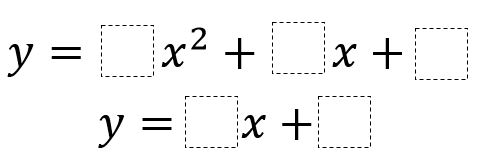# Line Tangent to a Parabola

Directions: Use the digits 1 to 9, at most one time each, to fill in the boxes so that the line is tangent to the parabola.### Hint

Set the functions equal to each other. When solving this equation, the discriminant must be 0.

### Answer

One possible answer:
y=x^2+6x+7
y=2x+3

Source: Erin Stenger

## Derivative of Trig Functions 2

Directions: Fill in the boxes below using the digits 1 to 6, at most one …